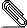# Re: [NTG-context] Placement of numbers in numbered equations on the left

```Hi Hans,

Following up the previous bug report, I tried the latest version, ConTeXt  ver:
2016.09.09 08:21 MKIV beta, and noticed that you have solved one part of the
problem with the placement of equation numbers on the left, when using
\startalign.
Actually the location of those equation numbers is correct as far as the
horizontal distance is concerned, but it is not vertically.
In the attached PDF (which is the result of the following example) one can see
that equation number (5) is placed on the grid line 10, while it shoul dbe
placed on line 11 in alignment with the corresponding formula. Moreover
equation number (4) does not show up at all (that number should be placed a
little bit below line 9 of the grid).```
```
Best regards: OK

%%% begin example bug-mathalign.tex
\showgrid

\starttext
With the default setting, that is \type{\setupformulas[location=right]} one
gets:
\placeformula
\startformula
\startalign
\NC \exp(t_1)\exp(t_2)\NC = x_1x_2=\exp(\log(x_1x_2))\NR[eq:explag0]
\NC \NC =\exp(\log x_1+\log x_2) = \exp(t_1+t_2).\NR[eq:explag1]
\stopalign
\stopformula

\placeformula
\startformula
1+1=2
\stopformula

\setupformulas[location=left]
While with \type{\setupformulas[location=left]} one gets:

\placeformula
\startformula
\startalign
\NC \exp(t_1)\exp(t_2)\NC = x_1x_2=\exp(\log(x_1x_2))\NR[eq:explag0]
\NC \NC =\exp(\log x_1+\log x_2) = \exp(t_1+t_2).\NR[eq:explag1]
\stopalign
\stopformula

\placeformula
\startformula
2+3=5
\stopformula
\stoptext
%%% begin example bug-mathalign.tex

```bug-mathalign-left.pdf

```

> On 7 Sep 2016, at 20:25, Otared Kavian <ota...@gmail.com> wrote:
>
> Hi Hans,
>
> Thank you for having fixed the issue with numbered equations in math aligned
> environment, noticed and reported by Mikael Sundqvist.
> However the problem is solved only when the numbers are on the right: when
> the numbers are on the left, there is still a discrepancy between the
> location of numbers in equations numbered in an aligned environment and those
> which are by themselves.
> Please have a look at the attached PDF which is the result of the following
> example (using ConTeXt  ver: 2016.09.06 19:11 MKIV beta).
> Best regards: OK
>
> %%% begin math-align.tex
> \starttext
> \placeformula
> \startformula
> \startalign
> \NC \exp(t_1)\exp(t_2)\NC = x_1x_2=\exp(\log(x_1x_2))\NR
> \NC \NC =\exp(\log x_1+\log x_2) = \exp(t_1+t_2).\NR[eq:explag1]
> \stopalign
> \stopformula
>
> \placeformula
> \startformula
> 1+1=2
> \stopformula
>
> \setupformulas[location=left]
> with \type{\setupformulas[location=left]}
>
> \placeformula
> \startformula
> \startalign
> \NC \exp(t_1)\exp(t_2)\NC = x_1x_2=\exp(\log(x_1x_2))\NR
> \NC \NC =\exp(\log x_1+\log x_2) = \exp(t_1+t_2).\NR[eq:explag1]
> \stopalign
> \stopformula
>
> \placeformula
> \startformula
> 2+3=5
> \stopformula
> \stoptext
> %%% end math-align.tex
>
> <math-align.pdf>
>
>

```
```___________________________________________________________________________________